Computer Science

# Lab 2c — Decomposition

## Preparation

### 5 minutes

Download the zip file for this lab, located above. This zip file has worlds that you will use for Bit. Extract the files and put them in your `cs110` directory in a folder called `lab2c`.

## Exercise 1

### 5 minutes

Bit starts in this world: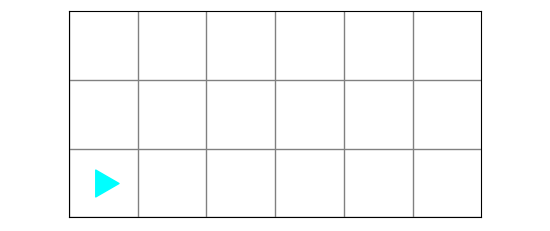and runs this code:

``````from byubit import Bit

def leap(bit):
if bit.front_clear():
bit.move()
if bit.front_clear():
bit.move()
if bit.front_clear():
bit.move()

def paint_spots(bit):
while bit.front_clear():
bit.paint('blue')
leap(bit)
bit.paint('blue')

def fill_green(bit):
while bit.front_clear():
bit.paint('green')
bit.move()
bit.paint('green')

@Bit.empty_world(6, 3)
def go(bit):
fill_green(bit)
bit.left()
bit.left()
paint_spots(bit)

if __name__ == '__main__':
go(Bit.new_bit)
``````

(1) What does the final world look like?

(2) In the code you downloaded, find `leap.py` and run it to be sure you have the right answer.

Discuss with the TA if there is anything you don’t understand about functions.

## Surround

### 15 minutes

Bit starts in a world that has some rectangular blocks. Here is world #1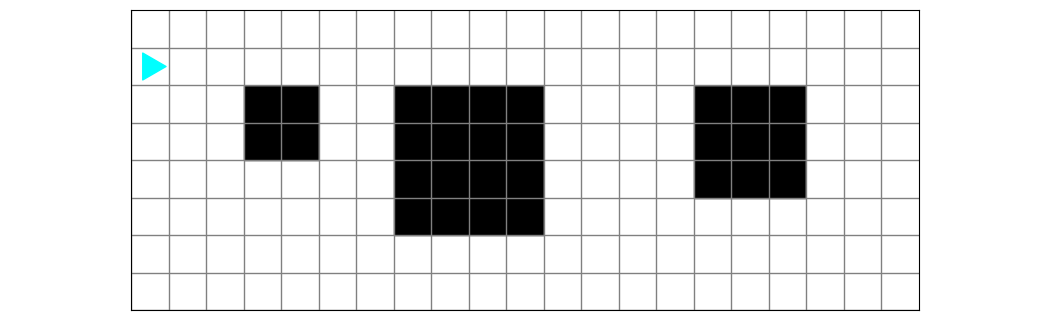and world #2:Bit needs to surround each of the blocks with green squares: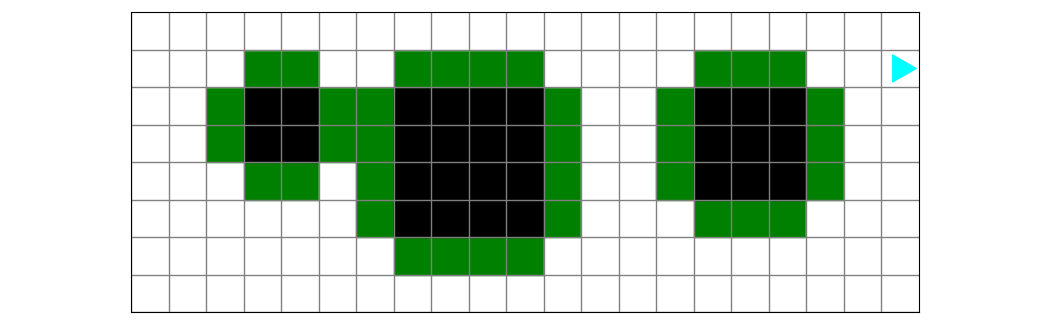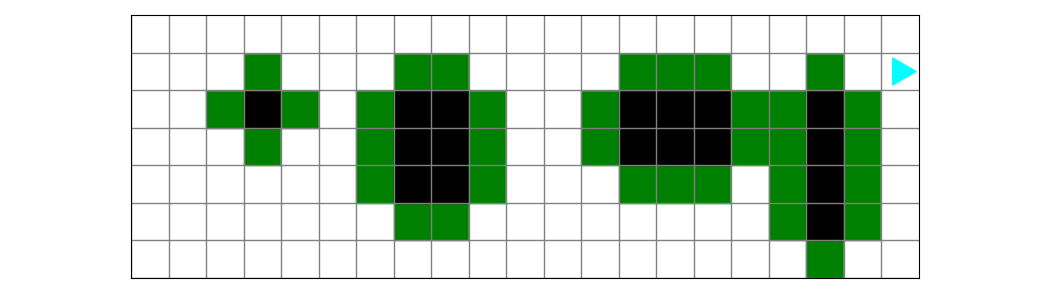1. This is a decomposition problem! Work with a friend to draw out your solution. See the guide on decomposition for examples of how to do this.

2. After you draw it out, discuss the steps with the TA.

3. Once you have a good idea of how to solve it, write code, using functions.

You can find starter code in `surround.py`.

## Blossoms

### 15 minutes

Bit starts in a world that has some empty pools. Here is world #1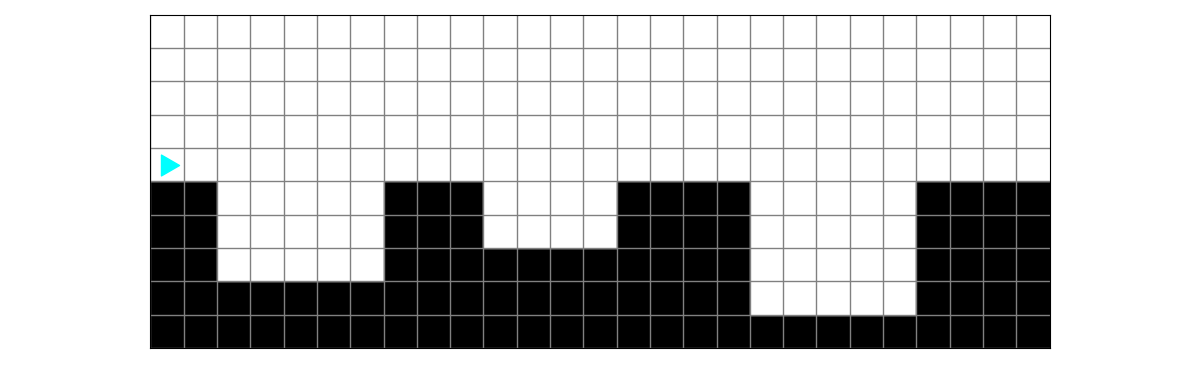and world #2: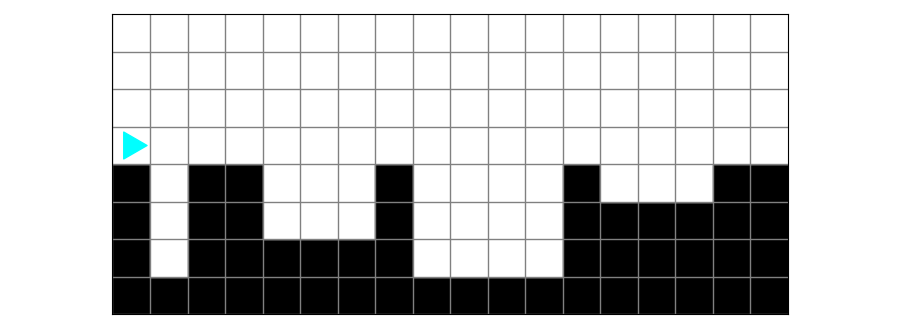Bit needs to fill each pool with water and then plant a flower on the right side: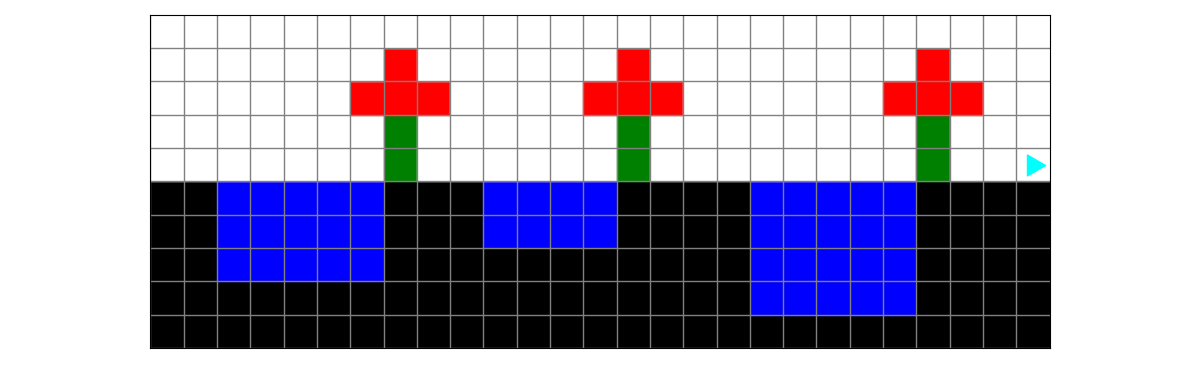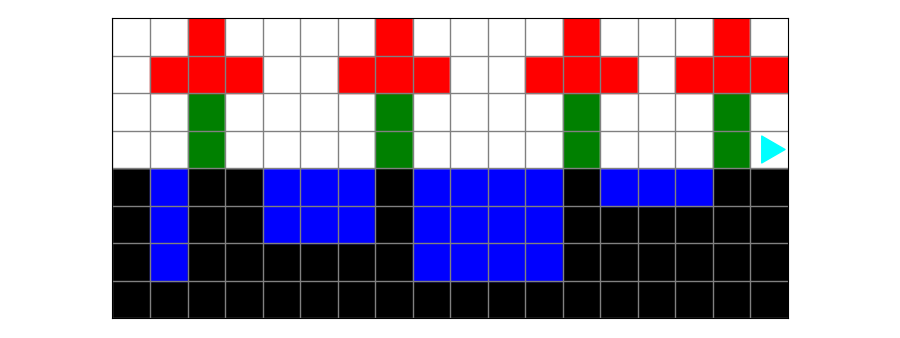1. Guess what, this is another decomposition problem! :-) Work with a friend to draw out your solution.

2. After you draw it out, discuss the steps with the TA.

3. Be careful about filling the pool. You can do this left to right or top to bottom.

4. Once you have a good idea of how to solve the problem, write code, using functions.

You can find starter code in `blossoms.py`.

## Preview Homework

### 10 minutes

Discuss the next set of homework problems. Are there any difficulties you anticipate? Talk about decomposition strategies, but each student should write their own code.